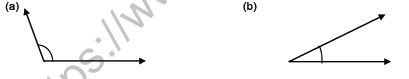# CBSE Class 5 Mathematics Question Paper Set W

Read and download CBSE Class 5 Mathematics Question Paper Set W designed as per the latest question paper pattern and Class 5 examination guidelines issued by CBSE, NCERT and KVS. The past year Question Papers for Class 5 Mathematics have been provided with solutions which will help students to assess their performance and find out topics in Mathematics grade 5 which they need to improve to get better marks in Standard 5 exams. After solving these last year papers also refer to solved Sample Papers for Class 5 Mathematics available on our website to build strong understanding of the subject

## Mathematics Question Paper Class 5

Students can refer to the below Class 5 Mathematics Question Paper designed to help students understand the pattern of questions that will be asked in Grade 5 exams. Please download CBSE Class 5 Mathematics Question Paper Set W

### Mathematics Class 5 Question Paper

Q.NO1

MCQ

(a) The greatest 5-digit number using the digits 6, 0, 7, 8, 2 is _________

a) 26780            b) 20678          c) 87602                 d) 87620

(b) If C.P =₹ 516 and Loss = ₹ 16, then S.P = ₹ ______ a) 500             b) 532                c) 166                 d) 32

(c) The sum of 5000 + 60 + 9 is __________ a) 5609      b) 5069         c) 5690               d) 5869

(d) The HCF of 5 and 16 is __________ a) 1                    b) 5               c) 16                  d) 80

(e) The number exactly divisible by 4 is ________ a) 233                      b) 600                c) 501                d) 405

FILL IN THE BLANKS

(f) If Dividend = 46327, divisor = 100 then Quotient = ______ and Remainder = _____

(g) The only even prime number is _____

(h) A triangle has _____ angles.

(i) The product of 7039 x 298 x 0 is ________

(j) 817325 + 308148 = __________ + 817325.

WRITE TRUE OR FALSE

(k) 698 rounded to the nearest 10 is 690

(l) If S.P = ₹ 8690 and Profit =₹ 650 then C.P is ₹ 8000

(m) If 2/5 = 16/x then x = 40

(n) 0 ÷ 6989 = not defined

(o) The vertex of /ABC is point B

COLUMN A COLUMN B

(p) Right angle                                      ( i ) 4820

(q) Roman numeral of 59                        ( ii ) 55/9

(r) Lowest form of  18/27                       ( iii) 90˚

(s) 241 × 20                                         ( iv) 2/3

(t) 6 1/9                                               ( v) LIX

Q.NO

(2) Draw the factor tree of 75

(3) 1kg of chocolates cost ₹ 559. Find the cost of 9 kg of chocolates?

(4) What must be added to 253107 to get 465000?

(5) Arrange the following in ascending order: 405623 ; 405263 ; 405326 ; 405632

(6) Check if the fractions 32/72  and 4/9 are equivalent or not.

(7) Identify the type of the angle